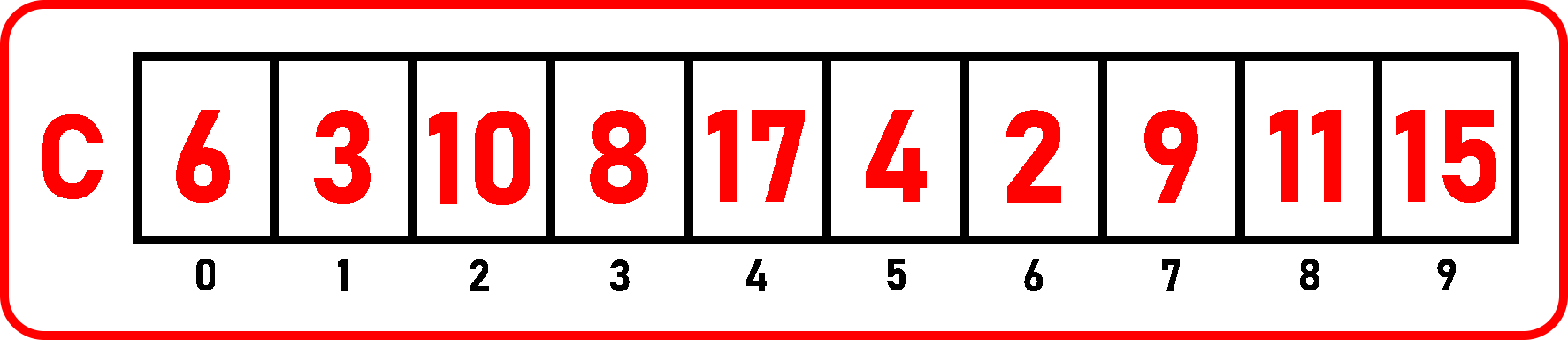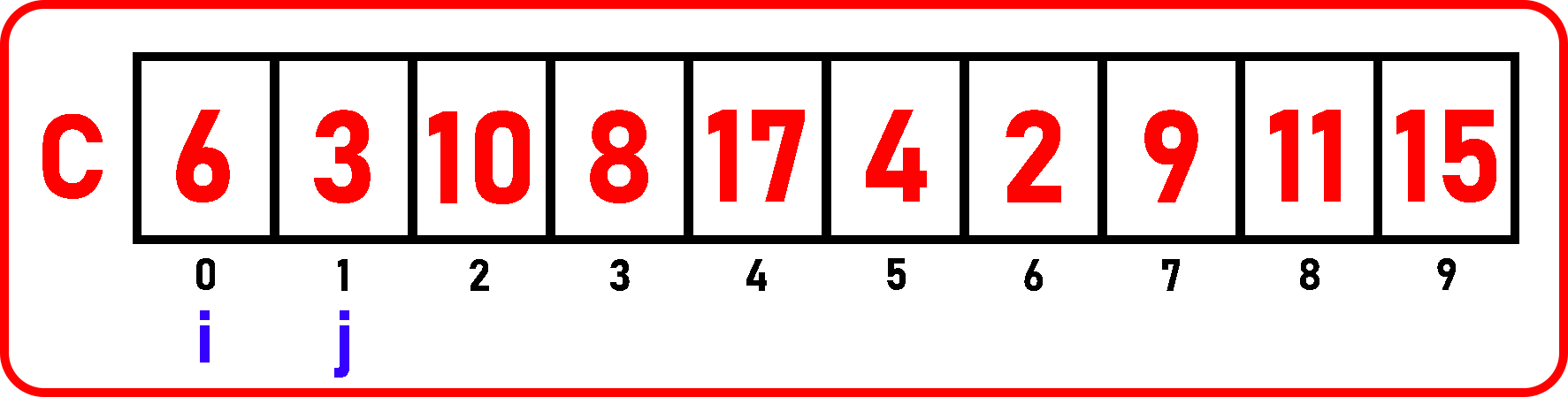# Finding a Pair of Element with Sum K from an Unsorted Array in C

## Finding a Pair of Element with Sum K from an Array in C Language:

In this article, I am going to discuss Finding a Pair of Element with Sum K from an Array in C Language with Examples. In our previous article, where we discussed Finding Duplicate Elements in an Unsorted Array in C Language.

##### Finding a Pair of Element with Sum K from an Array:

In our previous article, we have seen how to find duplicate elements in an unsorted array with and without hashing technique. In this article, we will see how to find a pair of elements with the sum ‘k’. If a list of elements is given, then we have to find out a pair of elements i.e. two elements such that their sum is equal to some given number:

A + B = 10

Let’s assume we want two elements A and B and such that the total is equal to 10.We have taken an array ‘C’ of size 10. We have to check in this array, are there any two elements whose sum is equal to 10? So, as we can directly see that there are total 2 pairs whose sum is equal to 10:

6 + 4 = 10,

8 + 2 = 10

So now we need an algorithm for our code that will produce the same result as we calculated directly. So, there can be several methods for finding that pairs but here we will cover two methods:

##### 1st Method to Find a Pair of Element with Sum K from an Array:

We have to scan the whole array, so we are taking a variable i initialized to 0th index and for checking another element we require one more variable, we call it as j and initialize it on j+1 position:j will check the rest of the array and find the element which will give i + j = 10. So, we will increment j and check each and every element with i and will print only those elements which will give the sum of 10. Now, i is pointing on 6 and j is pointing on 3. 6 and 3 aren’t correct pairs to get the sum of 10. So, we have to increment j.

Then j will point on 10, which is also not a correct element to give the sum of 10. Then j will point 8, 17. These aren’t giving the sum of 10. Again, increment j to the next element.j is pointing on 4 and 6 + 4 = 10. So j is pointing on the correct index. Here we will print the ith and jth elements. In this way, j will start from the i+1 position and will point to each and every element. So, we can say that j will check right-hand side elements because already i is pointing on the left-hand side elements.

Note: In this example, we have taken all unique elements. There is no duplicate of any element.

We have already discussed how to find and count the duplicate in our previous article. If there were duplicate elements, then first we have to remove those duplicates and then proceed to this step. Let if any duplicate is present in our array, then we check first and mark that duplicate as -1.

We are searching in following manner:

= n-1 + n-2 + n-3 + … + 3 + 2 +1

We have seen the same equation in finding duplicate elements and we know it is equal to:

= n (n-1) / 2

Time Complexity: O (n2)

##### Find a Pair of Element with Sum K from an Array Code in C language:
```#include <stdio.h>
#include <stdlib.h>
struct List{
int C;
int size;
int length;
};

void Display(struct List list) {
int i;
printf("Elements are:\n");
for (i = 0;i<list.length;i++)
printf("%d ", list.C[i]);
printf("\n\n");
}

void SumPairs(struct List list, int sum){
for(int i = 0; i < list.length - 1; i++){
for(int j = i + 1; j < list.length ; j++){
if(list.C[i] + list.C[j] == sum)
printf("%d + %d = %d\n", list.C[i], list.C[j], sum);
}
}
}

int main(){
struct List list_1 = {{6, 3, 10, 8, 17, 4, 2, 9, 11, 15}, 10, 10};
Display(list_1);
SumPairs(list_1, 10);
}
```
###### Output:##### 2nd Method to Find a Pair of Element with Sum K from an Array using Hash Table:

The previous method takes quadratic time so that was a time-consuming method. Let’s see another method for finding pairs for a given sum. In this method, we will use a hash table.As we know already that we will take the hash table of size equal to the largest element present in the given array. In our case, 17 is the largest element so we have taken the hash table of index 17 and initialized all the elements with zero. We will scan through the array as we have done in the previous method:As i is pointing on the 6, so what number we require to get the sum 10? 4, but 4 isn’t present in hast table as it is 0 there, here just increment the index 6 in the hash table:Now move i to the next element, as we can see in the array, 4 is the matching element which is present at the index of 5 in array ‘C’. So, we directly jump there assuming we have incremented those indices in the hash table as:Hash table is updated as:Now, i is on 4, what element should be added with 4 so that sum will be 10? The element is 6. Check if 6 is 1 or 0 in the hash table, yes 6 index is 1 means we have found a pair so now we have to print that pair on screen. In this way, our hashing method will work for finding pairs for the given sum.

##### Find a Pair of Element with Sum K from an Array Code in C language using Hash Table:
```#include <stdio.h>
#include <stdlib.h>
struct List{
int C;
int size;
int length;
};

void Display(struct List list) {
int i;
printf("Elements are:\n");
for (i = 0;i<list.length;i++)
printf("%d ", list.C[i]);
printf("\n\n");
}

void SumPairs(struct List list, int max, int sum){

int H[max];

for(int i = 0; i <= max; i++){
H[i] = 0;
}

for(int i = 0; i < list.length; i++){
if(H[sum - list.C[i]] != 0 && (sum - list.C[i] > 1)){
printf("%d + %d = %d\n", list.C[i], sum - list.C[i], sum);
}
H[list.C[i]]++;
}
}

int main(){
struct List list_1 = {{6, 3, 10, 8, 17, 4, 2, 9, 11, 15}, 10, 10};
Display(list_1);
SumPairs(list_1, 17, 10);
}
```

Time Complexity: O (n)

###### Output:In this article, I am going to discuss How to Find a Pair of Elements with Sum K from a sorted Array in C Language with Examples. Here, in this article, I try to explain How to Find a Pair of Elements with Sum K from an Array in C Language with Examples and I hope you enjoy this Finding a Pair of Elements with Sum K from an Array in C Language with Examples article.